## Recognizing Patterns:

Can you find the particular pattern to each of the sequences below? See if you can guess the number that comes next for each set of numbers below!

a) 1, 1, 1, 1, 1, ?
b) 1, 2, 3, 4, 5, ?
c) 2, 4, 6, 8, 10, ?
d) 5, 10, 15, 20, ?

Easy to recognize what comes next, right? But how did you know? What pattern did each example make? That’s what we’ll be talking about in this post, sequences!

## What is a Sequence?

Let’s start with a formal definition. A sequence is a list of numbers or objects that are in a particular order and form a pattern. Each example above is a different type of sequence that follows a specific pattern! That’s why we were able to easily find the successive term of each sequence (solution to each example below).

The above examples of sequences are just a snippet of what a sequence can look like, but there are so many sequences that exist! There is even a website that compiles every sequence in the world possible, called The On-Line Encyclopedia of Integer Sequences (OEIS), it’s like a kind of dictionary but for sequences! They also accept new sequences to their website. After reading this post, see if you can come up with your own sequence to add to their encyclopedia!

## Types of Sequences:

There are so many different types of sequences! Sequences can take so many different forms, they can be infinite sequence where they can go on forever, or they can be finite sequence where they have an end. A sequence can be based on addition, subtraction, multiplication, division, or even based on the value of the previous term! Let’s take a look at each type of sequence one step at a time with an example for each:

## Infinite Sequences

Infinite Geometric Series

## Special Sequences

Fibonacci Sequence
Summing Every number from 1 to 100

## Arithmetic Sequence:

Arithmetic sequences are a sequence of numbers that form a pattern when the same number is either added or subtracted to each successive term. Take a look at the example of the of the arithmetic sequence below. Notice we are adding 2 to each term in the sequence 4, 6, 8, 10, … below. The number we add to each term in this sequence (in this case 2), is called the common difference. If we were to find the next term in the example of the sequence below, the next term of the sequence would be 12 (10+2=12).

Sometimes, we are asked to find not just the next term, but a term further down the line in the sequence. For example, what if we were asked to find the value of the 123rd term? That’s where the arithmetic sequence formula would come in! Check out the explicit formula below:

an=a1+(n-1)d

a1=First Term
n=Term Number in Sequence
d=Common Difference (Number Added/Subtracted to each Term in Sequence)

Now, to find the 123rd term of the above sequence, we would plug in the following values into our formula to solve. Notice the common difference here is still 2, because it is the number we are adding to all the terms in our sequence.

Notice the above arithmetic sequence is a an example of an infinite sequence! This means that we can continue finding terms for this sequence forever!

Want another example? For more examples and a step-by-step video on arithmetic sequences, check out the resources here and below:

## Geometric Sequence:

Geometric sequences are a sequence of numbers that form a pattern when the same number is either multiplied or divided to each subsequent term. Take a look at the example of a geometric sequence 4, 8, 16, 32, … below. Notice we are multiplying 2 to each term in the sequence below. The number that is multiplied to each term in the sequence is called the common ratio, which in the case of the geometric sequence below is 2. If the pattern were to continue, the next term of the sequence above would be 64, since 32 x 2=64.

Sometimes, we are asked to find not just the next term, but a term far down the line in the sequence, for example, what if we were asked to find the value of the 15th term? That’s where the geometric sequence formula would come in!

an=a1r(n-1)

a1 = First Term
r=Common Ratio (Number Multiplied/Divided to each Term in Sequence)
n= Term Number in Sequence

To find the 15th term of the above sequence, we would need to plug in the following values into our formula to solve:

Notice the above geometric sequence is a type of infinite sequence! This means that we can continue finding terms for this sequence, forever!

Want another example? For more examples and a step-by-step video on geometric sequences, check out the resources here and below:

## Recursive Sequences:

A Recursive Formula is a type of formula that forms a sequence based on the previous term value. These can be based on arithmetic sequences or a geometric sequences, the sequence type does not really matter, as long as each successive term is based on the previous term. What does that mean? Check out the example below for a clearer picture:

What are the first 5 terms of a recursive sequence given the following recursive formula:

First, let’s decode what this says:

Now, let’s see our formula in action! Notice below, we start with the first term, a1=2. Then we use 2 to plug into our formula for the second term to get 2+4=6, and we continue the pattern all the way through for the first five terms, to get our answer.

Recursive Sequence: Now that have used our recursive formula, notice it gave us this nice, beautiful sequence represented by each term circled in pink above, 2, 6, 10, 14, 18. That is our recursive sequence, and the answer to our question! Notice in this example, we have an infinite sequence that we can keep finding terms for, but since our question asked for the first 5 terms, we stopped here.

Want another example? For more examples and a step-by-step video on recursive sequences, check out the resources here and below:

## Finite Sequence and Series:

Now that we have gone over infinite sequences (Arithmetic, Geometric, Recursive), let’s dive into finite sequence and series!

What’s the difference between a sequence and a series? We know what sequences are, but what is this new word “series” all about? Well, what if we had the first 5 terms of a sequence, but now, we want to add them all together? That is a series, more specifically a finite series since we only want to add the first five terms! Another term you might see that describes a finite sequence is “Partial Sum” or “Partial Sums” because we are summing only part of the sequence.

## Finite Arithmetic Series:

A finite arithmetic series happens when we take the terms of an arithmetic sequence and we sum a finite number of them together. Basically, we know that the arithmetic sequence gives us the following terms:

But now we want to sum all these terms together:

How would we be able to find the partial sum of the first 20 terms of an arithmetic sequence? Well, we could sit there and crunch our numbers one by one on a calculator, or we could plug them into the arithmetic finite sequence formula below:

Sn=(n/2)(2a1+(n-1)d)

n=Number of Terms we want to Sum

d=Common Difference

a1=First Term

To find the sum of the first 20 terms of the above sequence, we would need to plug in the following values into our formula to solve. Notice that in this case, the common difference is 2, since each subsequent term is being added by 2.

For more examples and a step-by-step video on how to find the finite length of a Finite Arithmetic Series, check out the resources here and below:

## Finite Geometric Series:

A finite geometric series happens when we take the terms of a geometric sequence, and we sum them together. Basically, we know that the geometric series gives us the following terms:

But now we want to sum all these terms together:

How would we be able to find the partial sums of the first 20 terms of a geometric sequence? Well, we could sit there and crunch our numbers one by one on a calculator, or we could plug them into the formula below:

Sn=a1 (1-rn)/(1-r)

a1=First Term

n=Number of Terms we are Summing together

r=Common Ratio

For more examples and a step-by-step video on finite sequences and Geometric Series, check out the resources here and below:

## Infinite Sequences:

An infinite sequence is one in which the sequence just keeps going and going infinitely with no end. We have already seen examples of this earlier in this post when looking at an arithmetic sequence or geometric sequence. But now, we want to ask ourselves, what would happen if we were to add an entire infinite sequence, by adding together each term within the sequence?

When it comes to adding an infinite arithmetic sequence together, the arithmetic sequence always diverges to infinity. On the other hand, an infinite sequence that is also a geometric sequence, can either diverge to infinity, or converge to a number. If the idea of converging or diverging to infinity, doesn’t make sense yet, that’s ok, keep reading and we’ll go over everything!

## Infinite Geometric Series:

An infinite geometric series happens when we take the terms of a geometric sequence, and we sum them together, all of them together, starting with the first term, going all the way to infinity. Basically, we know that the geometric series gives us the following terms:

But now we want to sum all these terms together, from the first term all the way to infinity.

But wait, how do we know if it is even possible to sum together a geometric sequence starting with the first term and adding until infinity? Well, it all depends on divergence vs. convergence. If the common ratio is between -1 and 1, that means that the sum to infinity will converge and we can find the value of the sum of an infinite geometric sequence. If the common ratio has any other value otherwise it will diverge to infinity.

*Also here is a reminder of what a geometric sequence looks like, for anyone who needs it!

Now, let’s see if the following geometric series converges or diverges:

Since, we know it converges based on the common ratio, we know we can find the value of the infinite sum! Let’s use the formula below:

Sn=a1/(1-r)

a1=First Term

r=Common ratio

Next, let’s plug in our value into the formula:

For more examples and a step-by-step video on Infinite Geometric Series, check out the resources here and below:

## Fibonacci Sequence – The Most Famous Sequence!

The above sequence, known as the “Fibonacci Sequence,” is the most famous sequence in the world! How can a sequence be so famous? Why is it so special? Well, we’ll get to that in a minute, but before we do, can you see how the Fibonacci Sequence forms a pattern? What would be the next term?

Take your time, trying to figure out the next term of the sequence before taking a peak at the answer below:

## Why!? Solution Explained

The next term of the Fibonacci sequence is 21! The pattern of this famous sequence is all about adding the two previous terms together. That’s how we get 1+1=2, 1+2=3, 2+3=5, 3+5=8, 5+8=13, which brings us to get our missing term, 8+13=21. Take a look at how this sequence works below:

## Why is the Fibonacci Sequence Famous?

Now that we know the secret pattern behind this sequence, let’s look at why the Fibonacci sequence is so special! The Fibonacci sequence’s main claim to fame is that it is found throughout art, architecture and even in nature via the golden ratio.

The Golden Ratio is a proportion that is considered to be the most pleasing ratio to the human eye! You may also know the Golden Ratio as the golden mean, the divine proportion, phi, or the Greek letter ϕ. It is an infinite and irrational number that approximates to 1.618 and is found by adding two numbers together and then dividing by the larger number and if these same two numbers are then set equal to the larger number divided by the smaller number successfully, then the two numbers are a golden ratio equal to 1.618! If this sounds too confusing to imagine, just take a look at the formula below:

## Is it a Golden Ratio?

If the following formula holds true, then yes!

What is amazing about this ratio, is that it can be related back to the Fibonacci Sequence!

## The Golden Ration + Fibonacci Sequence:

If we were to take the sequential numbers found within the Fibonacci sequence (1,1,2,3,5..), and plug them into the golden ratio formula above, it would approximate to the golden ratio value, 1.618, the further along in the sequence we go.

## The Golden Ratio + Art + Architecture + Nature:

If we were to draw a rectangle that has golden ratio proportions, we would get the golden rectangle below.

Let’s draw a golden rectangle, within our golden rectangle to see what happens:

What happens if we continue this specific pattern and keep drawing in golden rectangles within itself?

Until eventually we get something like this….

The proportion between the width and height of these rectangles is 1.618 and can also be shown as the proportion between any two numbers in the Fibonacci sequence as the sequence approaches infinity.

The above pattern, when all lines are connected form a spiral that can be found within art, architecture, and nature itself! Below we have a picture of the Parthenon, the Mona Lisa, a shell, and the Taj Mahal.

## The Golden Ratio + YOU:

Want to know if you yourself has a face that fits the golden ratio?! Try measuring your face horizontally and vertically and plug in those values into the golden ratio formula, dividing the larger number by the smaller number. What did you get? Something close to 1.6 maybe!?

## The Golden Ratio + Resources:

If you’re looking to learn more about the Golden Ratio, be sure to check out these resources here.

## Donald Duck’s – Mathmagic Land:

Video shows how the golden ratio works and where we can find it! https://www.youtube.com/watch?v=U_ZHsk0-eF0&t=1s

## Investopedia + Golden Ratio:

Learn how the Fibonacci Sequence connects to the modern-day stock market! https://www.investopedia.com/articles/technical/04/033104.asp

## The Golden Ratio + Mathematics:

Learn where you will see the Golden Ratio in mathematic equations and shapes!
https://www.mathsisfun.com/numbers/golden-ratio.html

## Summing Numbers from 1 to 100:

Now that we’ve discussed the most famous sequence, let’s talk about the most well-known sequence, which are just plain old-fashioned natural numbers, starting at 1 and counting to infinity! The sequence we all grew up with and learned to love, yes, natural numbers:

1,2,3,4,5, ….100, …..

What if we were tasked with summing all the numbers from 1 to 100? How would we do that? Well, believe or not, there is a formula to adding all of these numbers together, that was discovered by a famous mathematician named Johann Karl Friedrich Gauss. Apparently, he came up with this pattern after being assigned to add all the numbers from 1 to 100 as an elementary school student! The teacher thinking this would be perfect “busy” work for her class did not see it coming!

## How to Sum 1 to 100:

Gauss found a pattern, when adding together the numbers 1 to 100. He looked at the big picture, recognizing that the sum between pairs of numbers from the beginning of the sequence and at the end of the sequence were the same throughout the entire list of numbers! For example, 1+100=101, 2+99=101, 3+98=101, and this pattern continues between all the numbers from 1 to 100! Take a look:

## Formula and Solution:

Noticing this pattern, lead Gauss to come up with the following formula to sum every number from 1 to 100:

Above we use the formula to sum every number from 1 to 100 but it can be used to find other sums as well! If you’re wondering where in the world the above formula even came from, try testing it out with a smaller sum, summing numbers from 1 to 10 and see what happens!

Just like we find the sum in an arithmetic or geometric series, we can find the sum of other types of sequences, as we see here with natural numbers! We can even realize that summing numbers 1 to 100 is like summing an arithmetic sequence with a common difference of 1. Hidden patterns and formulas are used to solve all different types of sequences we haven’t even gone over in this post. But let this post guide you with the basics! See if you can find the pattern of any sequences that you find (in this post, in class, or in the street walking around, as sequences are found there too).

If you’re looking for more resources, check out this website that connects Gauss’s Summation formula to arithmetic sequences found in the real world here.

I hope you find this overview of sequences helpful! If there is anything you’d like me to go over more or if you have any questions, please let me know in the comments. Also, please check us out on social media for the latest MathSux videos, lessons, practice, questions, cheat sheets, and more! Happy calculating!

## 30 60 90 Triangle

Hi everyone and welcome to MathSux! In this post, we are going to break down 30 60 90 degree special right triangles. What is it? Where did it come from? What are the ratios of its side lengths and how do we use them? You will find all of the answers to these questions about this right angled triangle in this post. Also, don’t forget to check out the video below and practice questions at the end of this post to truly become a 30 60 90 special right triangle master! Happy calculating! 🙂

If you want to learn about the other special triangle, 45 45 90 triangle, check out this post here. And if you’re looking to make math suck just a little bit less? Subscribe to our Youtube channel for free math videos every week! 🙂

## 30 60 90 Triangle Ratio:

Notice that the 30 60 90 triangle is made up of one right angle across from the hypotenuse (which is always going to be the longest side), a 60 degree angle with a longer leg on the opposite side, and a 30 degree angle measure across from the shorter leg.

## What is a 30 60 90 Triangle and why is it “Special”?

The 30 60 90 triangle is a special right triangle because it forms an equilateral triangle when a mirror image of itself is drawn. This means that all sides are equal when a mirror image of the triangle is drawn which allows us to find the ratio between each of the sides of the triangle by using the Pythagorean Theorem. Check it out below!

Now let’s draw a mirror image of our triangle to create an equilateral triangle.  Next, we can label the length of the new side opposite 30 degrees (the shorter leg) “a,” and add this new mirror image length with the original we had to get, a total of a+a=2a.

If we look at our original 30 60 90 triangle, we now have the following values for each side based on our equilateral triangle. Notice we still need to find the length value of the longer leg, opposite angle 60 degrees.

To sum up, we have applied the Pythagorean Theorem formula, filled in values found in our triangle for each length, distributed the exponent and subtracted a2 from both sides, took the square root, and finally found our solution for our ratio for the longer leg opposite 60 degrees.

Now we can re-label our triangle, knowing the length of the hypotenuse in relation to the two legs. This creates a ratio that applies to all 30 60 90 triangles!

## How do I use this ratio?

Knowing the above ratio, allows us to find any length of any and every 30 60 90 triangle, when given the value of one of its sides. Let’s tsee how this ratio works with some examples.

## Example #1:

Step 1: First let’s look at our ratio and compare it to our given triangle.

Step 2: Notice we are given the value of a, which is equal to 4, knowing this we can now fill in each length of our triangle based on the ratio of a 30 60 90 triangle.

Now let’s look at another Example where we are given the length of the hypotenuse and need to find the values of the other two missing sides.

## Example #2:

Step 1: First let’s look at our ratio and compare it to our given triangle.

Step 2: Notice we are given the value of the hypotenuse, 2a=20. Knowing this we can find the value of a by dividing 20 by 2 to get a=10. Once we have the value of a=10, we can easily find the length of the last, longer leg based on the 30 60 90 ratio:

Now for our last Example, we will see how to find the value of the shorter leg and hypotenuse, when we are given the side length of the longer leg across from 60º and need to find the other two missing sides.

## Example #3:

Step 1: First let’s look at our ratio and compare it to our given triangle.

Step 2: In this case, we need to use little algebra to find the value of a, using the ratio for 30 60 90 triangles.

Now that we have one piece of the puzzle, the value of a, let’s fill in the value of the shorter leg of our triangle below:

Finally, let’s find the value of the length of the hypotenuse, which is equal to 2a in our ratio. Knowing the length of all sides, we can fill in the lengths of each side of our triangle for our solution.

Think you are ready to master these types of questions on your own? Try the practice problems below!

## Practice Questions:

Find the value of the missing sides of each 30 60 90 degree triangle.

## Solutions:

Still got questions? No problem! Don’t hesitate to comment with any questions or check out the video above. Happy calculating! 🙂

## Related Trigonometry Posts:

The Unit Circle

Basic Right Triangle Trigonometric Ratios (SOH CAH TOA)

4545 90 Special Triangles

Factoring Trigonometric Functions

Graphing Trigonometric Functions

Trig Identities

Transforming Trig Functions

Law of Cosines

Law of Sines

## Graphing Linear Inequalities: Algebra

graphing linear inequalities

Hi and welcome to MathSux! In this post, we are going to go over the rules for graphing linear inequalities on a coordinate plane when it comes to drawing lines, circles , and shading, then we are going to solve an example step by step. If you have any questions, check out the video below and try the practice questions at the end of this post! If you still have questions, don’t hesitate to comment below and happy calculating! 🙂

## Graphing Linear Inequalities:

When graphing linear inequalities, we always want to treat the inequality as an equation of a line in  form y=mx+b….with a few exceptions:

Now that we know the rules, of graphing inequalities, let’s take a look at an Example!

## Graphing Linear Inequalities Example:

Step 1: First, let’s identify what type of inequality we have here.  Since we are working with a > sign, we will need to use a dotted line and open circles when creating our graph.

Step 2: Now we are going to start graphing our linear inequality as a normal equation of a line, by identifying the slope and the y-intercept only this time keeping open circles in mind.  (For a review on how to graph regular equation of a line in y=mx+b form, click here)

Step 3: Now let’s connect our dots, by using a dotted line to represent our greater than sign.

Step 4: Now it is time for us to shade our graph, since this is an inequality, we need to show all of our potential solutions with shading.  Since we have a greater than sign, , we will be shading above the y-axis.  Notice all the positive y-values above are included to the left of our line.  This is where we will shade.

Step 5: Check!  Now we need to check our work.  To do that, we can choose any point within our shaded region, if the coordinate point we chose hold true when plugged into our inequality then we are correct!

Let’s take the point (-3,2) plugging it into our inequality where x=-3 and y=2.

## Solutions:

Still got questions? No problem! Don’t hesitate to comment with any questions or check out the video above for an in depth explanation. Happy calculating! 🙂

## How to Construct an Equilateral Triangle?: Geometry

Happy Wednesday math peeps! This post introduces constructions by showing us how to construct an equilateral triangle by using a compass and straightedge (or ruler). For anyone new to constructions in geometry, this is the perfect topic for art aficionados since there is more drawing here than there is actual math!

## What is an Equilateral Triangle?

Equilateral Triangle: An equilateral triangle is a triangle with three equal sides.  Not an easy one to forget, the equilateral triangle is super easy to construct given the right tools (compass+ straightedge). Take a look below:

## Construct an Equilateral Triangle Example:

Check out the GIF below to see how to construct an equilateral triangle step by step using a compass and straightedge with pictures and explanations below!

## What’s Happening in this GIF?

1. Using a compass, we measure the distance of line segment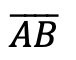.

2. With the compass point remaining on point A, we then draw an arc that has the same distance as line segment.

3. With the compass now placed on point B, draw an arc that has the same distance as line segment.

4. Notice where the arcs intersect? Using a ruler, connect points A and B to the new intersection point. This will create two new equal sides of our triangle!

5. We have now officially constructed a triangle with all equal side lengths!

## Constructions and Related Posts:

Looking to construct more than just an equilateral triangle? Check out these related posts on geometry constructions!

Construct a Perpendicular Bisector

Perpendicular Line through a Point

Angle Bisector

Construct a 45º angle

Altitudes of a Triangle (Acute, Obtuse, Right)

Construct a Square inscribed in a Circle

## Best Geometry Tools!

Looking to get the best construction tools? Any compass and straight-edge will do the trick, but personally, I prefer to use my favorite mini math toolbox from Staedler. Stadler has a geometry math set that comes with a mini ruler, compass, protractor, and eraser in a nice travel-sized pack that is perfect for students on the go and for keeping everything organized….did I mention it’s only \$7.99 on Amazon?! This is the same set I use for every construction video in this post. Check out the link below and let me know what you think!

Still got questions? No problem! Don’t hesitate to comment with any questions. Happy calculating! 🙂

Looking to learn more about triangles? Check out this post on right triangle trigonometry here! And if you’re looking for even more geometry constructions, check out the link here!

## Expanding Cubed Binomials: Algebra 2/Trig.

Greetings math friends! This post will go over expanding cubed binomials using two different methods to get the same answer. We’re so used to seeing squared binomials such as,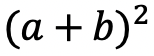, and expanding them without a second thought.  But what happens when our reliable squared binomials are now raised to the third power, such as,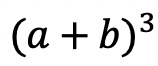?  Luckily for us, there is a Rule we can use:

But where did this rule come from?  And how can we so blindly trust it? In this post we will prove why the above rule works for expanding cubed binomials using 2 different methods:

Why bother? Proving this rule will allow us to expand and simplify any cubic binomial given to us in the future! And since we are proving it 2 different ways, you can choose the method that best works for you.

## Method #1: The Box Method

Step 1: First, focus on the left side of the equation by expanding (a+b)3:

Step 2: Now we are going to create our first box, multiplying (a+b)(a+b). Notice we put each term of (a+b) on either side of the box. Then multiplied each term where they meet.

Step 3: Combine like terms ab and ab, then add each term together to get a2+2ab+b2.

Step 4: Multiply (a2+2ab+b2)(a+b) making a bigger box to include each term.

Step 5: Now combine like terms (2a2b and a2b) and (2ab2 and ab2), then add each term together and get our answer: a3+3a2b+3ab2+b3.

## Method #2: The Distribution Method

Let’s expand the cubed binomial using the distribution method step by step below:

Now that we’ve gone over 2 different methods of cubic binomial expansion, try the following practice questions on your own using your favorite method!

Practice Questions: Expand and simplify the following.

Solutions:

Still, got questions?  No problem! Check out the video above or comment below! Happy calculating! 🙂

**Bonus: Test your skills with this Regents question on!

## Recursive Rule

Welcome to Mathsux! This post is going to show you everything you need to know about how to use a Recursive Formula by looking at three different examples of a recursive rule. Check out the video below for more of an explanation and test your skills with the practice questions at the bottom of this page.  Please let me know if you have any questions in the comments section below and happy calculating! 🙂

## What is a Recursive Formula or a Recursive Rule?

A Recursive Formula is a type of formula that forms a sequence based on the previous term value.  The recursive rules for each formula vary, but we are always given the first term and a formula to find the consecutive terms in the recursive sequence.

Recursive formula can be written as an arithmetic sequence (a sequence where the same number is either added or subtracted to each subsequent term to form a pattern) and recursive formulas can also be written as arithmetic sequences (a sequence where the same number is either multiplied or divided to each subsequent term to form a pattern). We’ll go over an example of each but both types of recursive rules are treated the same exact way!

What does all of this mean?  Check out the example below for a clearer picture.

## Example #1: Arithmetic Recursive Sequence

Step 1: First, let’s decode what these formulas are saying.

Step 2: The first term, represented by a1, is and will always be given to us. In this case, our first term has the value a1=2 and represents the first term of our recursive sequence.

a1= First Term=2

Step 3: We then plug in the value of our first term, which is a1=2 into our formula an+4 to get 2+4=6. The number 6 now has the value of our second term in the recursive sequence.

a1= 2 First Term

a2= (2)+4=6 Second Term

Step 4: Now we are going to continue the pattern, plugging in the value of each previous term to find the next consecutive terms in our recursive sequence.

The pattern can be more easily seen below. Notice we are able to find the value of all 5 terms of the recursive sequence for the solution only given the first term and recursive formula at the beginning of our question.

Step 5: We found the recursive sequence we were looking for: 2, 6, 10, 14, 18. Since the question was originally only asking for the value of the fifth term we know our solution only needs to be the value of the fifth term which is 18.

## Example #2: Geometric Recursive Sequence

Step 1: First, let’s decode what these formulas are saying.

Step 2: The first term, represented by a1, is and will always be given to us. In this case, our first term has the value a1=1 and represents the first term of our recursive sequence.

a1= First Term=1

Step 3: We then plug in the value of our first term, which is a1=1 into our formula 2an+1 to get 21+1=3. The number 3 now has the value of our second term in the recursive sequence.

a1= 1 First Term

a2= 2(1)+1=3 Second Term

Step 4: Now we are going to continue the pattern, plugging in the value of each previous term to find the next term in our recursive sequence.

The pattern can be more easily seen below. Notice we are able to find the value of all 3 terms of the recursive sequence for the solution only given the first term and recursive formula at the beginning of our question.

***Note this was written in a different notation but is solved in the exact same way! This recursive formula is a geometric sequence.

Step 5: We found the recursive sequence we were looking for: 1,3,9. Since the question was originally only asking for the value of the third term we know our solution only needs to be the value of the third term which is 9.

## Example #3:

Step 1: First, let’s decode what these formulas are saying.

Step 2: The first term, represented by a1, is and will always be given to us. In this case, our first term has the value a1=4 and represents the first term of our recursive sequence.

a1= First Term=4

Step 3: We then plug in the value of our first term, which is a1=4 into our formula 3an-1-2 to get 3(2)-1=5. The number 5 now has the value of our second term in the recursive sequence.

a1= 4 First Term

a2= 3(2)-1=5 Second Term

Step 4: Now we are going to continue the pattern, plugging in the value of each previous term to find the next term in our recursive sequence.

The pattern can be more easily seen below. Notice we are able to find the value of all 3 terms of the recursive sequence for the solution only given the first term and recursive formula at the beginning of our question.

Step 5: We found the recursive sequence we were looking for: 4,10,28. Since the question was originally only asking for the value of the third term we know our solution only needs to be the value of the third term which is 28.

Think you are ready to solve a recursive equation on your own?! Try finding the specific term in each given recursive function below:

## Related Posts:

Looking to learn more about sequences? You’ve come to the right place! Check out these sequence resources and posts below. Personally, I recommend looking at the arithmetic sequence or geometric sequence posts next!

Arithmetic Sequence

Geometric Sequence

Finite Arithmetic Series

Finite Geometric Series

Infinite Geometric Series

Golden Ratio in the Real World

Fibonacci Sequence

Still, got questions? No problem! Don’t hesitate to comment below or reach out via email. And if you would like to see more MathSux content, please help support us by following ad subscribing to one of our platforms. Thanks so much for stopping by and happy calculating!

***Bonus! Want to test yourself with a similar NYS Regents question on Recursive Formulas?  Click here! And if you want to learn about even more sequences, check out the link here!

## Reflections: Geometry

Greetings and welcome to Mathsux! Today we are going to go over reflections geometry, one of the many types of transformations that come up in geometry. And thankfully, reflection transformation is one of the easiest types to master, especially if you’re more of a visual learner/artistic type person. In this post, we will go over a reflection across the line x=-2, but if you want to see a reflection across the x axis, the y axis, and the line y=x, please check out the math video below! So let’s get to it! And if you’re new to transformations, check out translations here.

## What are Reflections?

A Reflection on a coordinate plane is exactly what you think! A reflection is a type of transformation in geometry where we reflect a point, a line segment, or a shape over a line to create a mirror image of itself.  Think of the wings of a butterfly, a page being folded in half, or anywhere else where there is perfect symmetry, each of these are great examples of a reflection!

## Reflection Rules:

If you are completely new to reflections, you may want to check out the video above that goes into deriving reflection rules over the different axis. But for those of you more comfortable with reflection, check out the example below where we reflect the image of a triangle onto itself over the line x=-2.

## Example:

Step 1: First, let’s draw in line x=-2. Note that whenever we have x equal to a number, we end up drawing a vertical line at that point on the x axis, in this case at x=-2.

Step 2: Find the distance each point is from the line x=-2 and reflect it on the other side, measuring the same distance.

First, let’s look at point C, notice it’s 1 unit away from the line x=-2 on the right. To reflect point c, we are going to count 1 unit but this time to the left of the line x=-2 and label our new point, C|.

Step 3: Now we are going to reflect coordinate point A in much the same way! Notice that point A is 2 units away on the left of line x=-2, we then want to measure 2 units to the right of our line x=-2 and mark our new coordinate point, A|.

Step 4: Lastly, we want to reflect coordinate point B. This time, point B is 1 unit away on the right side of the line x=-2, we then measure 1 unit to the opposite direction of our line, x=-2, and mark our new point, B|.

Step 5: Now that we have all the newly reflected coordinate points of our triangle, finally, we can now connect them all, for our fully reflected image of right triangle A|B|C|.

Notice our newly reflected triangle is not just a mirror image of itself, but when the original figure is reflected it actually ends up overlapping onto itself!? How did this happen? That is because this our reflection line came right down the middle of our original image, triangle ABC. Shapes that reflect onto themselves are a bit tricky but not impossible, just remember to measure out the distance of each coordinate point and reflections should be a breeze!

## Rigid Motion:

Reflections are a special type of transformation in geometry that maintains rigid motion, meaning when a point, line, or shape is reflected the angles, and line segments retain their value. For example, if we were to measure the area of both right triangles, before and after reflection, we would find the areas to remain unchanged. Meaning the area of triangle ABC is equal to the area of triangle A|B|C| . Another rigid transformation includes rotations and translations.

Looking to practice your new reflection skills? Try the reflection practice problems below, with solutions to each question, to truly master the topic! Happy calculating!

## Solutions:

Still got questions?  No problem! Check out the video above or comment below! There is also a bonus video if you scroll all the way down at the end of this post for anyone who wants to see how to reflect a line over the line x=2. See how it differs and how it is similar to the example shown here. Happy calculating! 🙂

Don’t forget to follow MathSux on social media for the latest tips and tricks to ace math!

And if you are looking for more Transformations Check out the related posts below and let me know if you have any questions? Or maybe you just want to share your favorite type of transformation? Either way, happy calculating!

Translations

Dilations

Rotation

## Piecewise Functions: Algebra

Greetings, today’s post is for those in need of a piecewise functions review!  This will cover how to graph each part of that oh so intimidating piecewise functions.  There’s x’s, there are commas, there are inequalities, oh my! We’ll figure out what’s going on here and graph each part of the piecewise-function one step at a time.  Then check yourself with the practice questions at the end of this post. Happy calculating! 🙂

## What are Piece-Wise Functions?

Exactly what they sound like! A function that has multiple pieces or parts of a function.  Notice our function below has different pieces/parts to it.  There are different lines within, each with their own domain.

Now let’s look again at how to solve our example, solving step by step:

Translation: We are going to graph the line f(x)=x+1 for the domain where x > 0

To make sure all our x-values are greater than or equal to zero, we create a table plugging in x-values greater than or equal to zero into the first part of our function, x+1.  Then plot the coordinate points x and y on our graph.

Translation: We are going to graph the line  f(x)=x-3 for the domain where x < 0.

To make sure all our x-values are less than zero, let’s create a table plugging in negative x-values values leading up to zero into the second part of our function, x-3.  Then plot the coordinate points x and y on our graph.

## Practice Questions:

Graph each piecewise function:

## Solutions:

Still got questions?  No problem! Check out the video above or comment below for any questions. Happy calculating! 🙂

***Bonus! Want to test yourself with a similar NYS Regents question on piecewise functions?  Click here.

## Intersecting Secants Theorem: Geometry

Ahoy! Today we’re going to cover the Intersecting Secants Theorem!  If you forgot what a secant is in the first place, don’t worry because all it is a line that goes through a circle.  Not so scary right? I was never scared of lines that go through circles before, no reason to start now.

If you have any questions about anything here, don’t hesitate to comment below and check out my video for more of an explanation. Stay positive math peeps and happy calculating! 🙂

Wait, what are Secants?

Intersecting Secants Theorem: When secants intersect an amazing thing happens! Their line segments are in proportion, meaning we can use something called the Intersecting Secants Theorem to find missing line segments.  Check it out below:

Let’s now see how we can apply the intersecting Secants Theorem to find missing length.

Step 1: First, let’s write our formula for Intersecting Secants.

Step 2: Now fill in our formulas with the given values and simplify.

Step 3: All we have to do now is solve for x! I use the product.sum method here, but choose the factoring method that best works for you!

Step 4: Since we have to reject one of our answers, that leaves us with our one and only solution x=2.

Practice Questions: Find the value of the missing line segments x.

Solutions:

Still got questions?  No problem! Check out the video above or comment below for any questions and follow for the latest MathSux posts. Happy calculating! 🙂

To review a similar NYS Regents question check out this post here.

## Circle Theorems & Formulas

Inscribed Angles & Intercepted Arcs

Area of a Sector

Circle Theorems

## Absolute Value Equations: Algebra

Happy Wednesday math friends! Today, we’re going to go over how to solve absolute value equations.  Solving for absolute value equations supplies us with the magic of two potential answers since absolute value is measured by the distance from zero.  And if this sounds confusing, fear not, because everything is explained below!

Also, if you have any questions about anything here, don’t hesitate to comment. Happy calculating! 🙂

Absolute Value measures the “absolute value” or absolute distance from zero.  For example, the absolute value of 4 is 4 and the absolute value of -4 is also 4.  Take a look at the number line below for a clearer picture: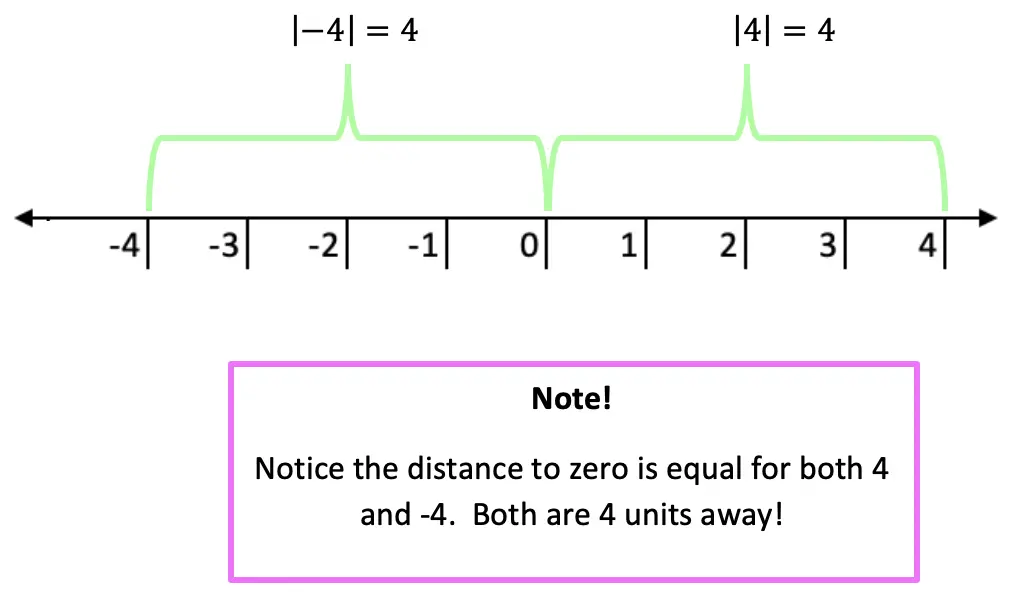Now let’s see how we can apply our knowledge of absolute value equations when there is a missing variable!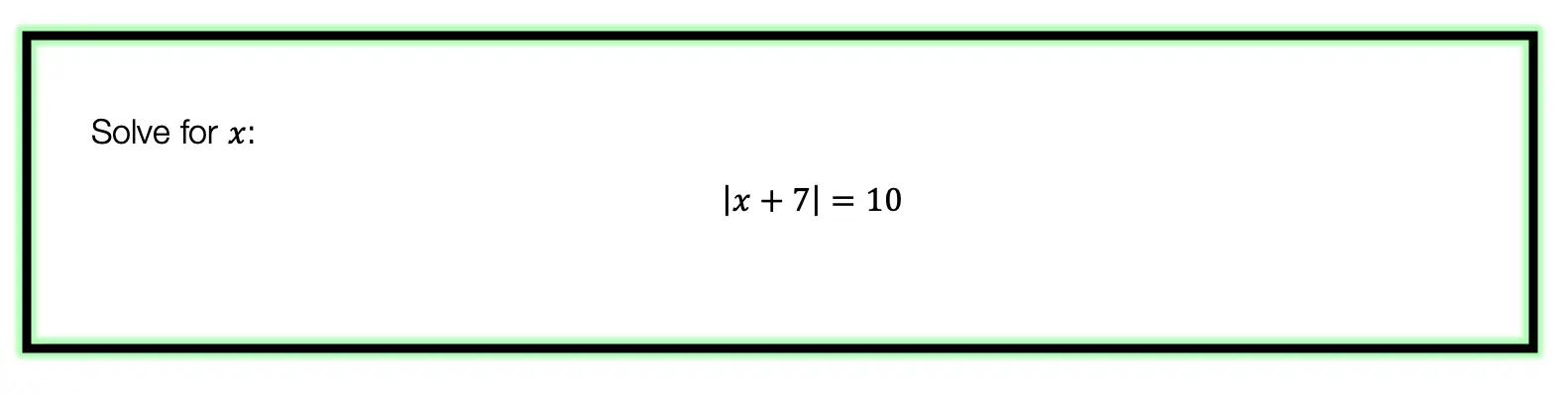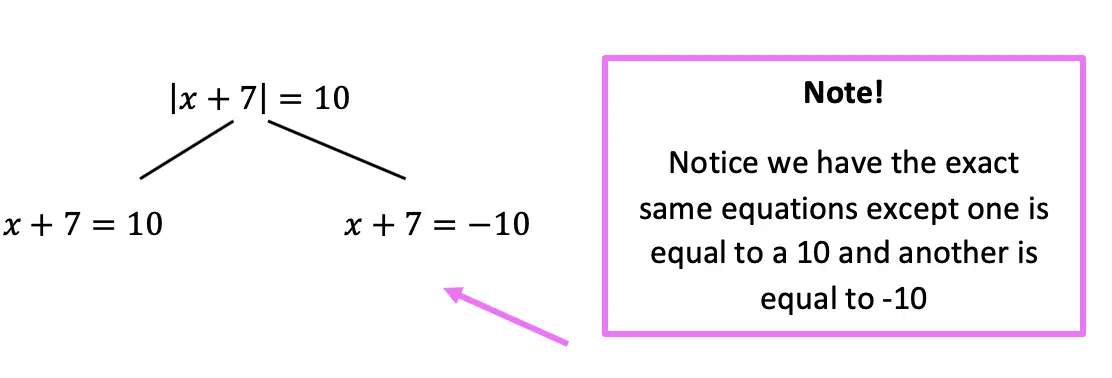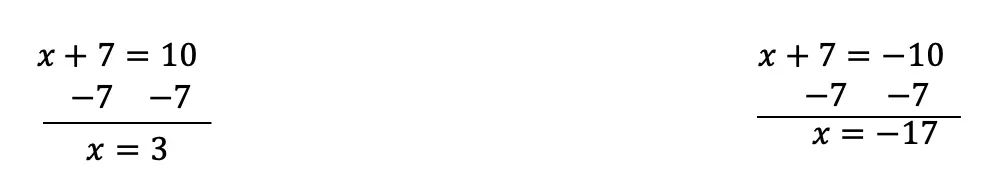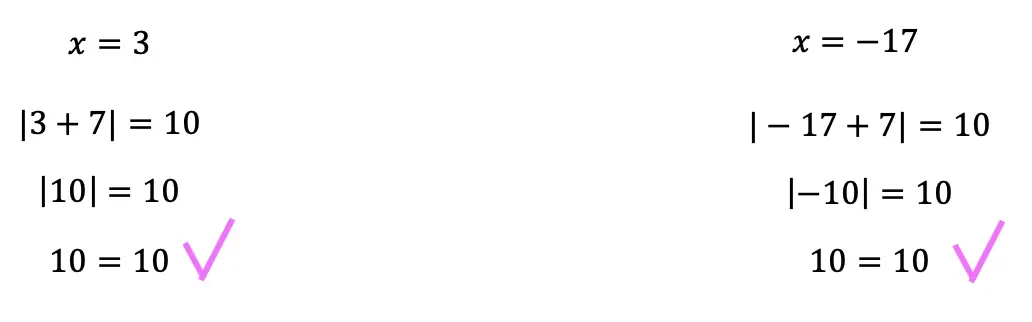Now let’s look at a slightly different example: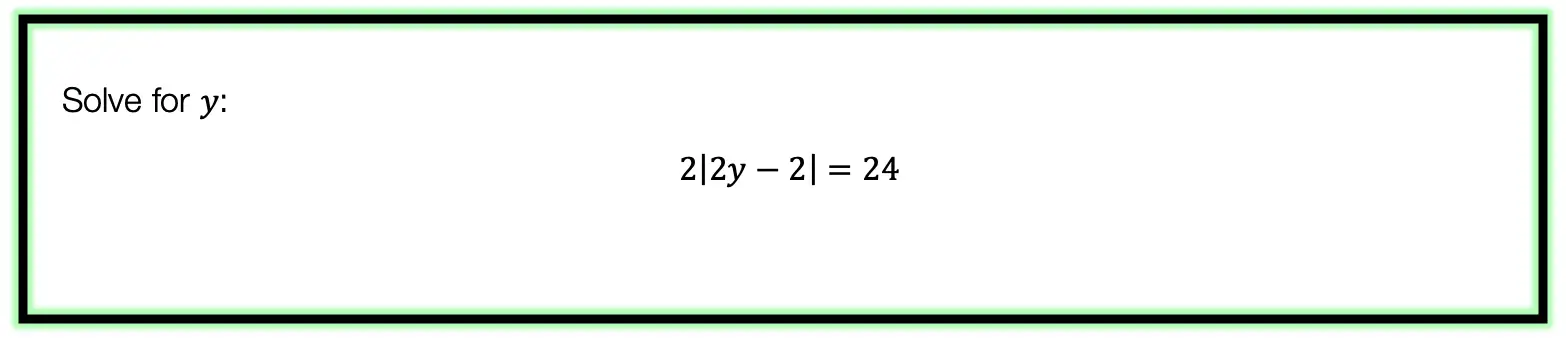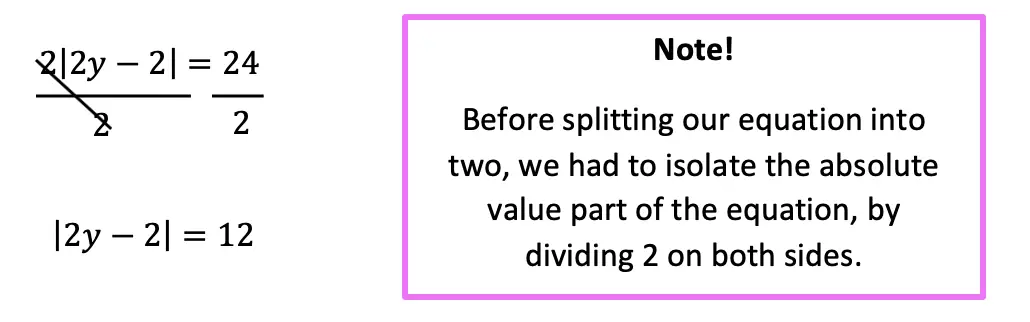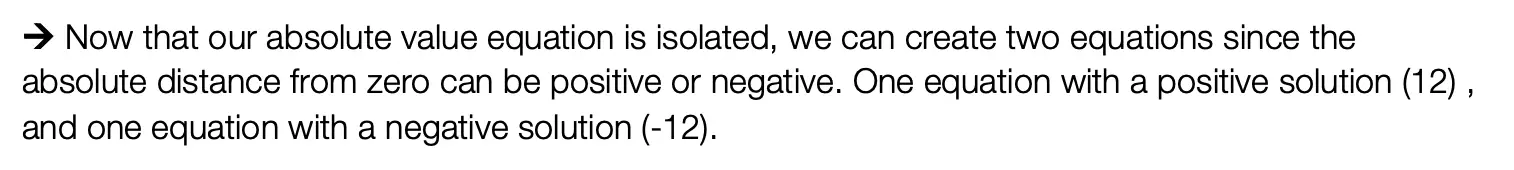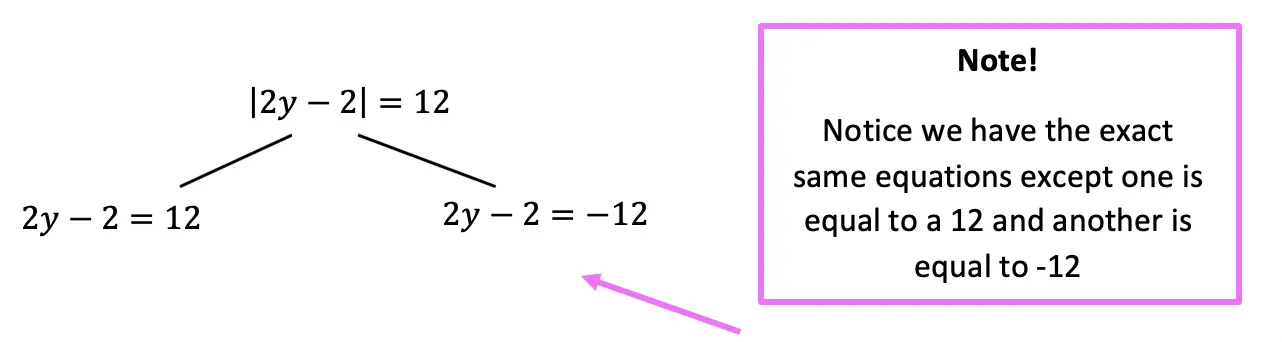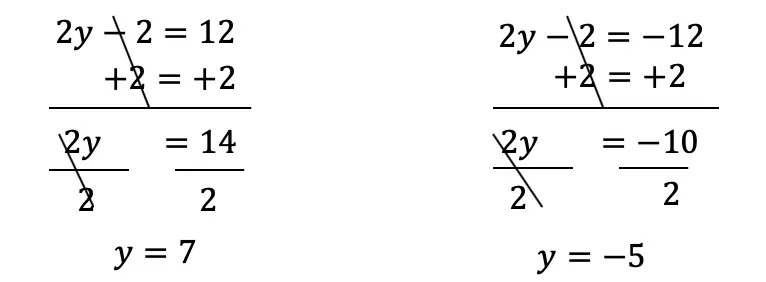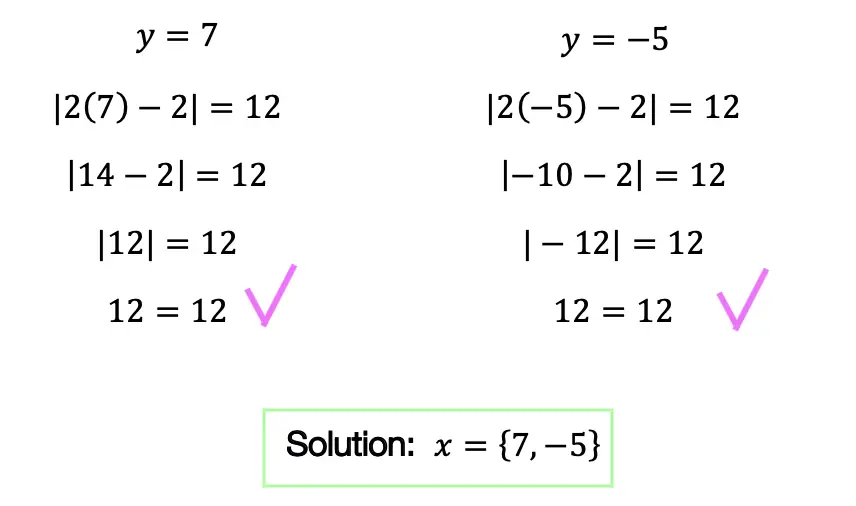Practice Questions: Given the following right triangles, find the missing lengths and side angles rounding to the nearest whole number.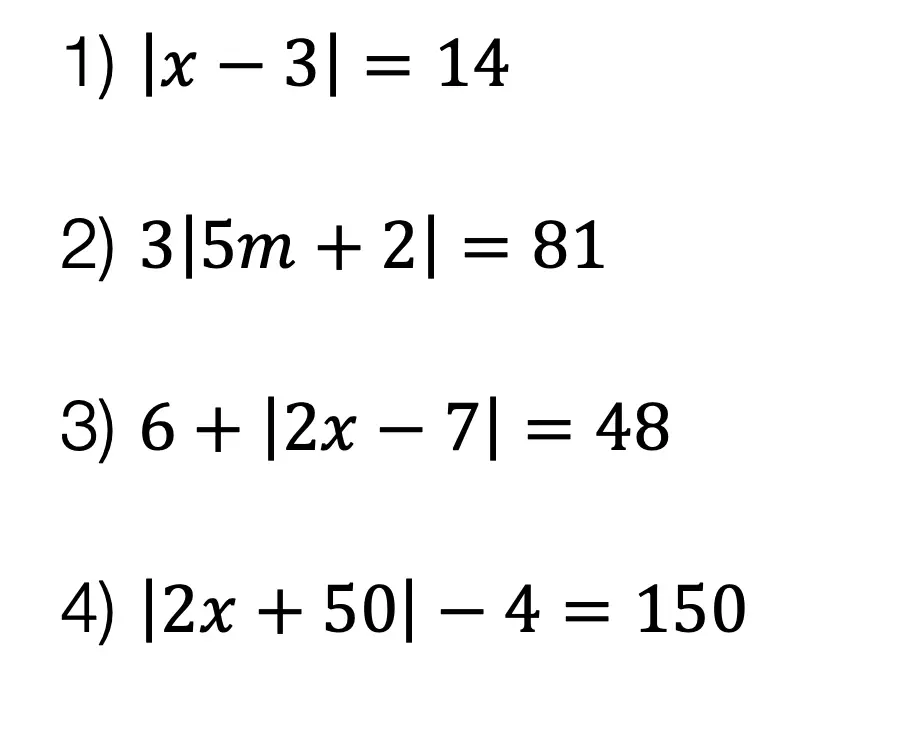Solutions: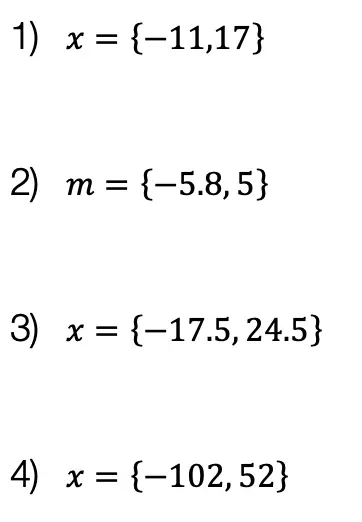Still got questions?  No problem! Check out the video the same examples outlined above. Happy calculating! 🙂

Also, if you’re looking for a review on combining like terms and the distributive property, check out this post here.